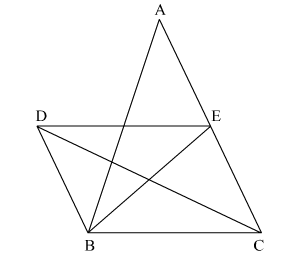# In the adjoining figure, BD || CA, E is the midpoint of CA and BD

Question:

In the adjoining figure, $B D \| C A, E$ is the midpoint of $C A$ and $B D=\frac{1}{2} C A$. Prove that $\operatorname{ar}(\triangle A B C)=2 \operatorname{ar}(\Delta D B C)$.Solution:

E is the midpoint of CA.
So, AE = EC                            .....(1)

Also, $\mathrm{BD}=\frac{1}{2} \mathrm{CA}$          (Given)

So, BD = AE                            .....(2)
From (1) and (2) we have
BD = EC
BD || CA and BD = EC so, BDEC is a parallelogram

$B E$ acts as the median of $\triangle A B C$

so, $\operatorname{ar}(\triangle \mathrm{BCE})=\operatorname{ar}(\triangle \mathrm{ABE})=\frac{1}{2} \operatorname{ar}(\triangle \mathrm{ABC})$   .......(i)

$\operatorname{ar}(\triangle D B C)=\operatorname{ar}(\triangle B C E)$         .....(2)                  (Triangles on the same base and between the same parallels are equal in area)

From (1) and (2)
ar(∆ABC) = 2ar(∆DBC)